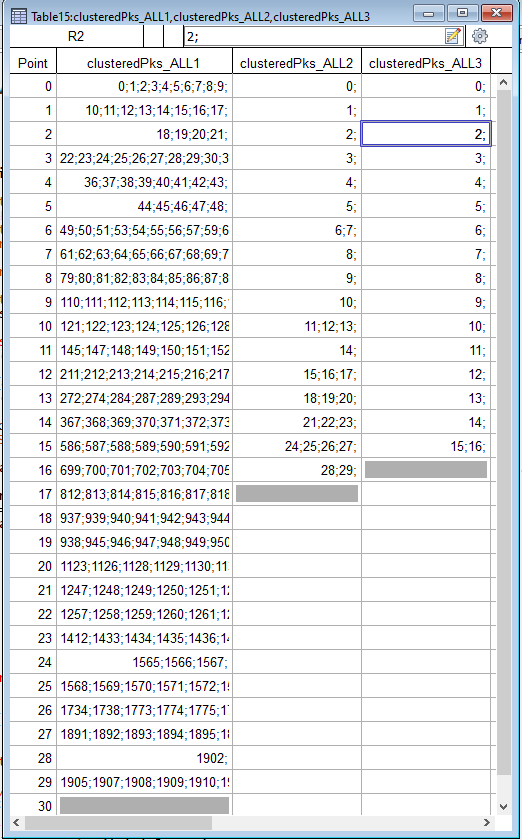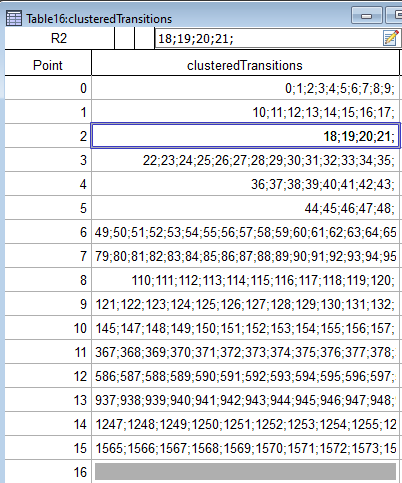# Generating a list from different text wavesHi there,

I'm working on a routine that is supposed to consolidate the results of a clustering process done over a few iterations in IGOR into a single text wave.

I start the process by creating a 2D wave that contains the percent overlap between a set of peaks in my data. Based on this overlap wave I then have a routine that determines whether the peaks get clustered together if their overlap exceeds some threshold value specified by the used. The percent overlap matrix between the generated clusters is created and the clustering process is repeated until the overlap matrix does not have any values above the threshold value.

For each iteration, a text wave is created where each point in the wave is a list of peaks contained in a cluster. This is where I'm having a bit of a problem. For the results of the first iteration, the text wave contains the raw/original list of peaks from my data. However, for the subsequent iterations the text wave is based on the clusters generated from the previous iteration. So there's a bit of a disconnect.

Here's an example of the text waves I'm mentioning:The three waves named clusteredPeaks_All1,clusteredPeaks_All2,and clusteredPeaks_All3 represent the text waves done at each clustering iteration. Here, clusteredPeaks_All1 represents the first clustering iteration that is done on the original set of peaks while clusteredPeaks_All3 is the final clustering iteration and represents that final set of clusters. The way to interpret is that the first clustering iteration resulted in a total of 30 clusters where the first cluster [point 0 in clusteredPeaks_All1] is made up of peaks 0;1;2;3;4;5;6;7;8;9; , the second cluster [point 1 in clusteredPeaks_All1] is made up of peaks 10;11;12;13;14;15;16;17; and so on and so forth. The second clustering iteration took the set of 30 peaks from the first iteration, determined their overlap, and based on that it determined that those 30 peaks could be reduced into a set of 17 peaks where the first cluster [point 0 in clusteredPeaks_All2] is made of cluster 0 from clusteredPeaks_All1 and is therefore also made up of peaks 0;1;2;3;4;5;6;7;8;9;. Lastly, the third and final iteration determined that the 17 clusters generated from the second iteration could be reduced down to 16 clusters where the first cluster [point 0 in clusteredPeaks_All3] is made up of cluster 0 from clusteredPeaks_All2 and is thus made up of peaks 0;1;2;3;4;5;6;7;8;9; and so on and so forth. That is what I would like to represent in my final result wave. I want the wave to show what peaks the final clusters are made from.

I made a routine that got me close to that but it's not quite right yet as shown here:The results from the clustering are placed into a text wave called clusteredTransitions that has as many points as the number of clusters from the final iteration. Here, cluster 0 [point 0] is made up peaks 0;1;2;3;4;5;6;7;8;9; and so on and so forth. The problem is that the last cluster does not catch the final set of peaks [points 28 and 29] from clusteredPeaks_All1.

Here's the routine that I've been trying to use to get this working:

Function consolidateTransitionClusters()

String cPkList = WaveList("clusteredPks_ALL*",";","")//List of clustering iteration waves
Variable nw = ItemsInList(cPkList)
String finClsName = StringFromList(nw-1,cPkList)//Final clusters
Wave/T wF = \$finClsName
String iniClsName = StringFromList(0,cPkList)//Initial clusters
Wave/T wI = \$iniClsName
Variable nFinCls = numpnts(wF),i,j,k,l,m=0,cpk //nFinCls defines final number of clusters
Make/O/T/N=(nFinCls) clusteredTransitions =""

for(i=1;i<nw;i+=1)//Choose cluster iteration wave
String ccw = StringFromList(i,cPkList)
Wave/T cw = \$ccw
Variable n = numpnts(cw)
for(j=0;j<n;j+=1)//Choose the current cluster
Variable nPeaks = ItemsInList(cw[j])
for(k=0;k<nPeaks;k+=1)
cpk = str2num(StringFromList(k,cw[j]))
if(i==1)
clusteredTransitions[m] += wI[cpk]//Is this the problem??
else
String ccwIni = StringFromList(i-1,cPkList)
Wave/T cwP = \$ccwIni
clusteredTransitions[m]+= wI[cpk]
endif
endfor
m+=1
if(m>=nFinCls)
m = 0
break
endif
endfor
//  m=0
endfor
//Remove duplicates that may be present within each cluster
for(i=0;i<nFinCls;i+=1)
clusteredTransitions[i] = SortList(clusteredTransitions[i],";",34)
endfor

//Check for multiple instances of same transitions within different clusters
i=0;j=0
for(i=0;i<nFinCls;i+=1)//Select initial transition cluster
String iniCluster = clusteredTransitions[i]
Variable nIni = ItemsInList(iniCluster)
for(j=0;j<nIni;j+=1)//Select transition in initial cluster to look for
String cPeak1 = StringFromList(j,iniCluster)
if(i!=k)
for(k=i+1;k<nFinCls;k+=1)//Select next transition cluster
String nextCluster = clusteredTransitions[k]
Variable nCur = ItemsInList(nextCluster)
for(l=0;l<nCur;l+=1)//Check for preexisting transitions in cluster
String cPeak2 = StringFromList(l,nextCluster)
if(Stringmatch(cPeak2,cPeak1))
clusteredTransitions[k] = RemoveFromList(cPeak2,clusteredTransitions[k])
endif
endfor
endfor
else
k+=1
endif
endfor
endfor
End

I've attached an IGOR file with the relevant waves and procedure.

Thanks for the help!!

do you get maybe an index out of range error?

I could imagine a different approach: say you have a wave containing the peak numbers and sets of clusters (e.g. 3 and 2) in the following way:

Make/O/N=20 Peaks = p

Make/O/N=(20,3) Clu1 = NaN
Clu1[0,4] = 1
Clu1[5,11] = 1
Clu1[12,19] = 1

Make/O/N=(20,2) Clu2 = NaN
Clu2[0,11] = 1
Clu2[12,19] = 1

Then you can use simple matrix calculations to get the peak numbers in the final clusters.